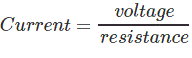# Measurement of Voltage Current and Frequency by Oscilloscope

Normally, an oscilloscope is an important tool in an electrical field which is used to display the graph of an electrical signal as it varies with respect to time. But some of the scopes have additional features apart from their fundamental use. Many oscilloscopes have the measurement tool that help us to measure waveform characteristics like frequency, voltage, amplitude, and many more features with accuracy. Generally, a scope can measure time-based as well as voltage-based characteristics.

## Voltage Measurement

The oscilloscope is mainly voltage oriented device or we can say that it is a voltage measuring device. Voltage, current and resistance all are internally related to each other.

Just measure the voltage, rest of the values is obtained by calculation. Voltage is the amount of electric potential between two points in a circuit. It is measured from peak-to-peak amplitude which measures the absolute difference between the maximum point of signal and its minimum point of the signal. The scope displays exactly the maximum and minimum voltage of the signal received. After measuring all high and low voltage points, scope calculates the average of the minimum and maximum voltage. But you must be careful to mention which voltage you mean. Normally, oscilloscope has fixed input range, but this can be easily increased with the use of simple potential divider circuit.

### Method to Measure Voltage

1. The simplest way to measure signal is to set the trigger button to auto that means oscilloscope start to measure the voltage signal by identifying the zero voltage point or peak voltage by itself. As any of these two points identified the oscilloscope triggers and measure the range of the voltage signal.
2. Vertical and horizontal controls are adjusted so that the displayed image of the sine wave is clear and stable. Now take measurements along the center vertical line which has the smallest divisions. Reading of the voltage signal will be given by vertical control.

## Current Measurement

Electrical current cannot be measured directly by an oscilloscope. However, it could be measured indirectly within scope by attaching probes or resistors. Resistor measures the voltage across the points and then substituting the value of voltage and resistance in Ohm’s law and calculates the value of electrical current. Another easy way to measure current is to use a clamp-on current probe with an oscilloscope.

### Method to Measure Current

1. Attach a probe with the resistor to an electrical circuit. Make sure that resistor’s power rating should be equal or greater than the power output of the system.
2. Now take the value of resistance and plug into Ohm’s Law to calculate the current.
3. According to Ohm’s Law,## Frequency Measurement

Frequency can be measured on an oscilloscope by investigating the frequency spectrum of a signal on the screen and making a small calculation. Frequency is defined as the several times a cycle of an observed wave takes up in a second. The maximum frequency of a scope can measure may vary but it always in the 100’s of MHz range. To check the performance of response of signals in a circuit, scope measures the rise and fall time of the wave.

### Method to Measure Frequency

1. Increase the vertical sensitivity to get the clear picture of the wave on the screen without chopping any of its amplitude off.
2. Now adjust the sweep rate in such a way that screen displays a more than one but less than two complete cycles of the wave.
3. Now count the number of divisions of one complete cycle on the graticule from start to end.
4. Now take horizontal sweep rate and multiply it with the number of units that you counted for a cycle. It will give you the period of the wave. The period is the number of seconds each repeating waveform takes. With the help of period, you can simply calculate the frequency in cycles per second (Hertz).

Want To Learn Faster? 🎓
Get electrical articles delivered to your inbox every week.
No credit card required—it’s 100% free.